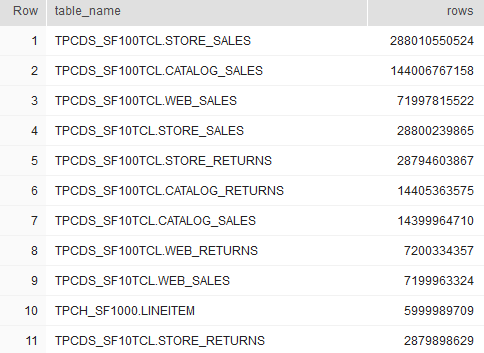## List tables by the number of rows in Snowflake

Article for:

This query returns list of tables in a database with their number of rows.

## Query

``````select t.table_schema || '.' ||  t.table_name as "table_name",
t.row_count as "rows"
from information_schema.tables t
where t.table_type = 'BASE TABLE'
order by t.row_count desc;
``````

## Columns

• table_name - table name with schema name
• rows - number of rows in a table

## Rows

• One row represents one table
• Scope of rows: all tables in a database including tables without rows
• Ordered by number of rows descending, from largest to smallest (in terms of number of rows)

## Sample results

Tables by number of rows in SNOWFLAKE_SAMPLE_DATA database :